# Assignments

In this series of assignments you get familiarized with trigonometric functions such as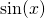,and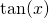. The angles are measured either in degrees or radians.
There are also  a few assignments about the unit circle and some relations between the various trigonometric functions.
Finally we know that some trigonometric functions can be used to demonstrate some trigonometric relations.

1. The following angles are given in degrees. Calculate them in radians.
30, 45, 90, 60, 180, 210, 225, 540

Solution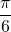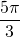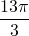Solution

3. Calculate using the table with special angles: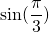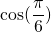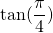Solution

4. Show using the unit circle: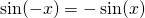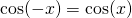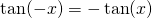Solution

5. Show using the unit circle: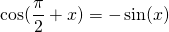Solution

6. Show using the unit circle: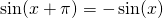Solution

7. Give the period of: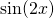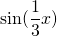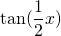Solution

8. Show: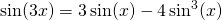Solution

9. Show: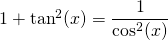Solution

10. Show for which values ofin the interval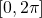the function: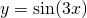takes the value.

Solution

0